# You Can Get 1Z0-808 Updated Dumps To Pass Java SE 8 Programmer I Exam

Are you preparing for Oracle 1Z0-808 Java SE 8 Programmer I exam for your Oracle Certified Associate, Java SE 8 Programmer Certification? Good news for you, you can get 1Z0-808 updated dumps to pass your Java SE 8 Programmer I exam. We have updated 1Z0-808 exam dumps questions recently, which came with 181 real exam questions and answers. We ensure that you can pass 1Z0-808 Java SE 8 Programmer I exam in the first try.

## Check Oracle 1Z0-808 Free Dumps Online

1. Given: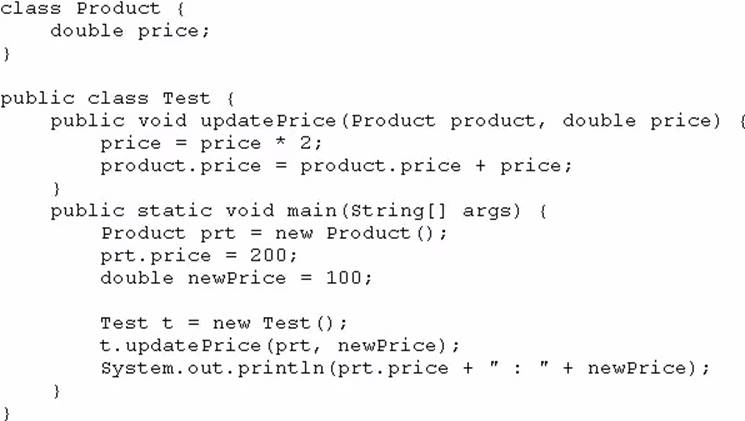What is the result?

2. Which statement is true about the switch statement?

3. Given the code fragment: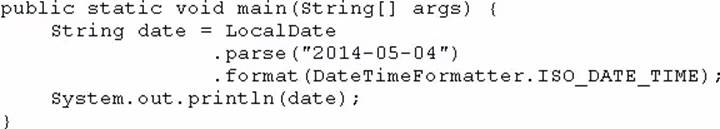What is the result?

4. Given the code fragment: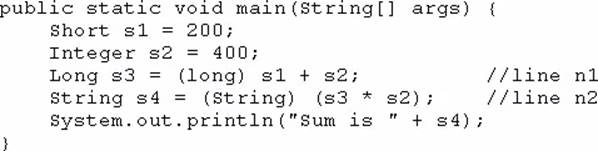What is the result?

5. What is the name of the Java concept that uses access modifiers to protect variables and hide them within a class?

6. Given the code fragment: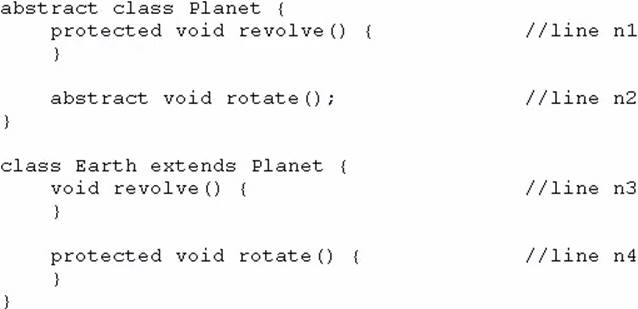Which two modifications, made independently, enable the code to compile? (Choose two.)

7. Given: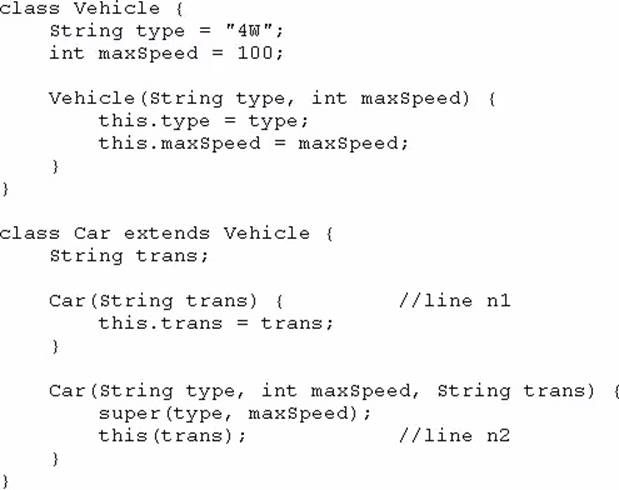And given the code fragment: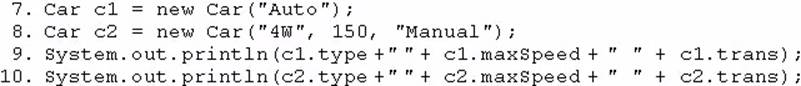What is the result?

8. Given: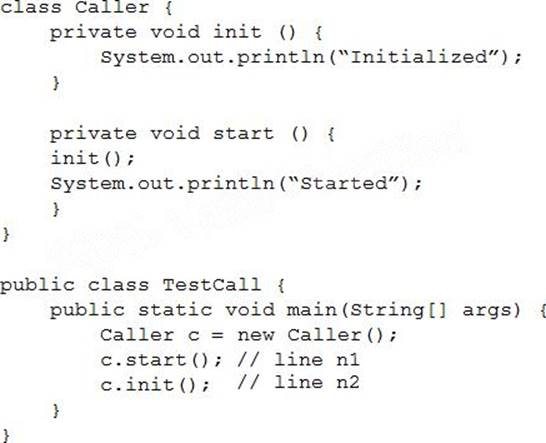What is the result?

9. Given these two classes: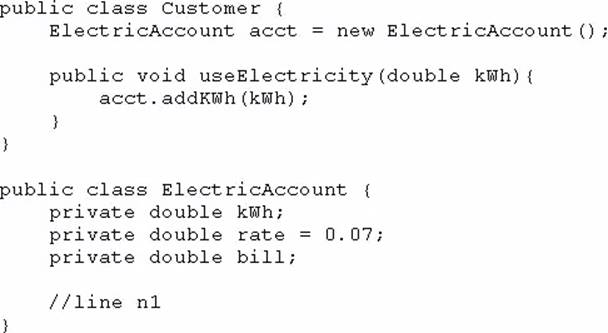Any amount of electricity used by a customer (represented by an instance of the Customer class) must contribute to the customer’s bill (represented by the member variable bill) through the useElectricity method.

An instance of the Customer class should never be able to tamper with or decrease the value of the member variable bill.

How should you write methods in the ElectricAccount class at line n1 so that the member variable bill is always equal to the value of the member variable kwh multiplied by the member variable rate?

A)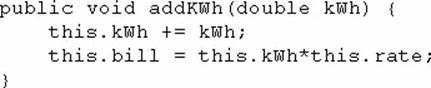B)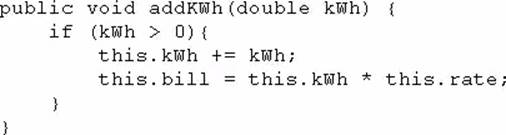C)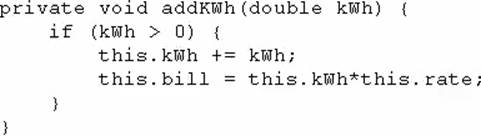D)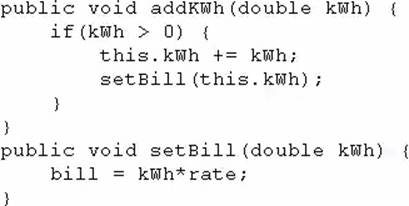10. Given the code fragment: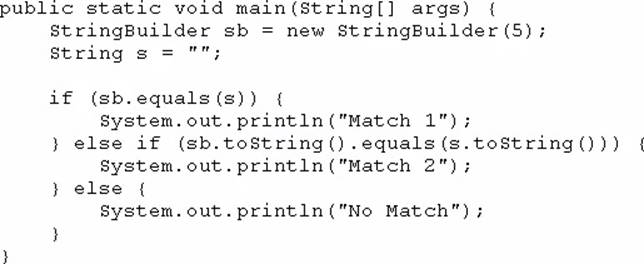What is the result?

11. Given: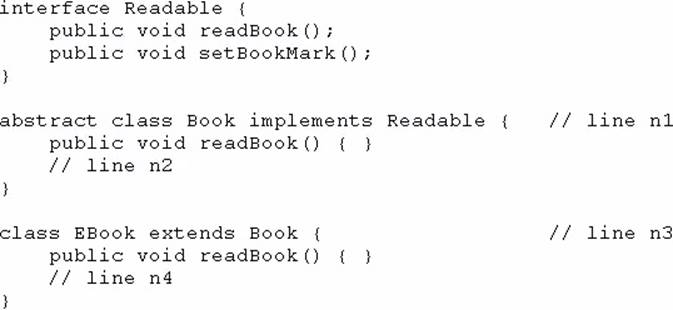And given the code fragment:

Book book1 = new EBook ();

Which option enables the code to compile?12. Given: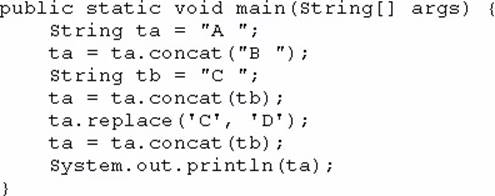What is the result?

13. Given: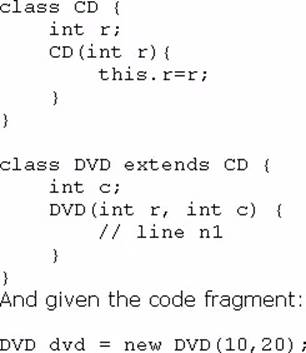Which code fragment should you use at line n1 to instantiate the dvd object successfully?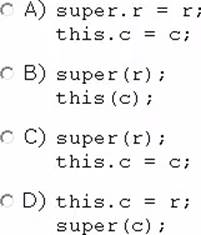14. Given the code fragment: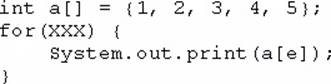Which option can replace xxx to enable the code to print 135?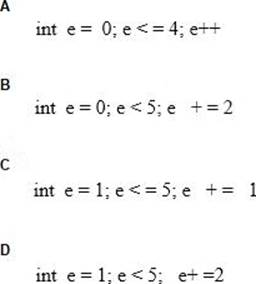15. Which statement best describes encapsulation?

16. Given the code fragment from three files: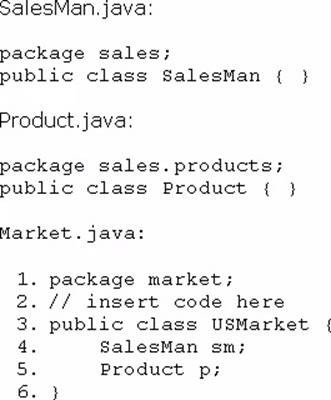Which code fragment, when inserted at line 2, enables the code to compile?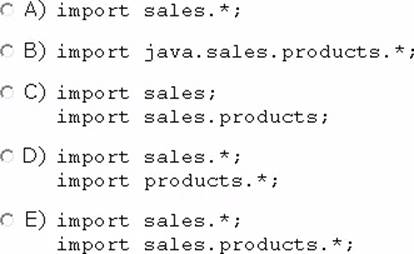17. Given this class: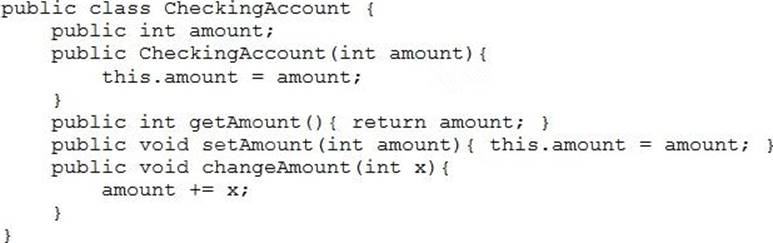And given this main method, located in another class: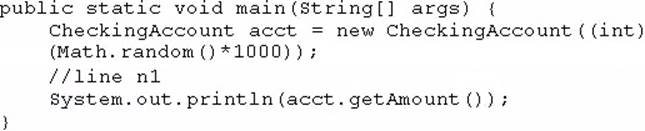Which three lines, when inserted independently at line n1, cause the program to print a 0 balance?

18. Given the code fragment: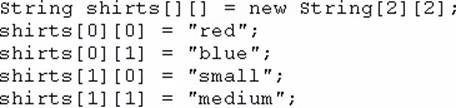Which code fragment prints red: blue: small: medium?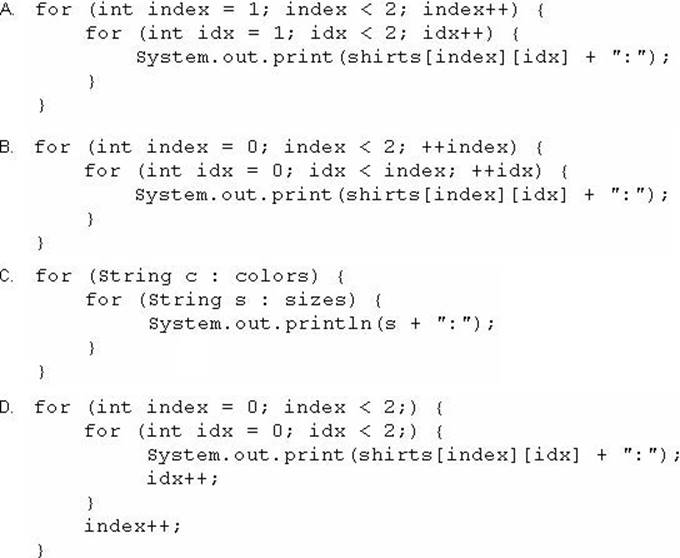19. Given the code fragment: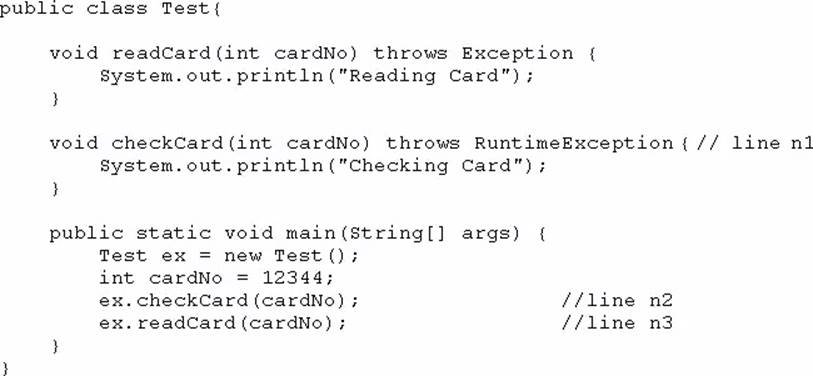What is the result?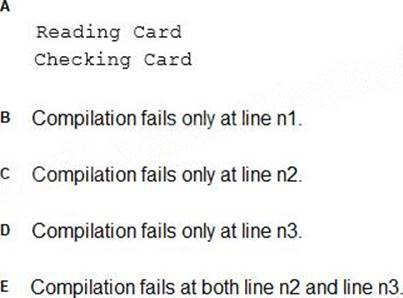20. Given the code fragment: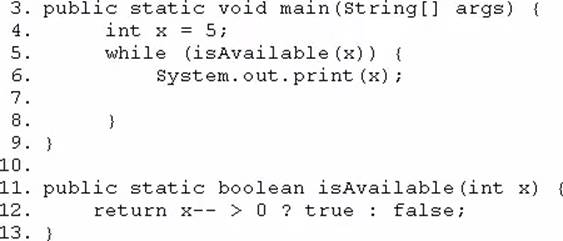Which modification enables the code to print 54321?

21. Given the code fragment: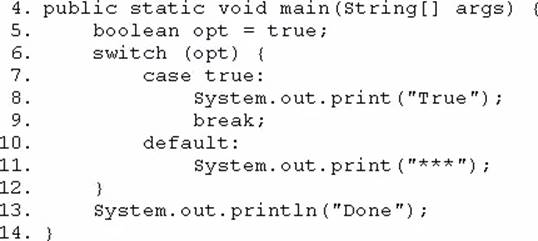Which modification enables the code fragment to print TrueDone?

22. Given the following main method: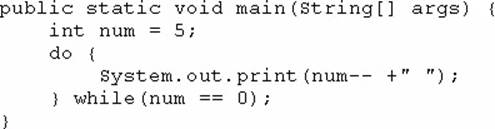What is the result?

23. Given the code fragment: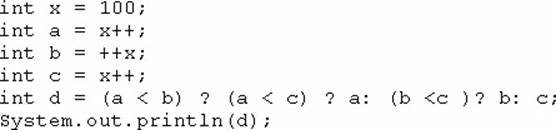What is the result?

24. Given: What is the result?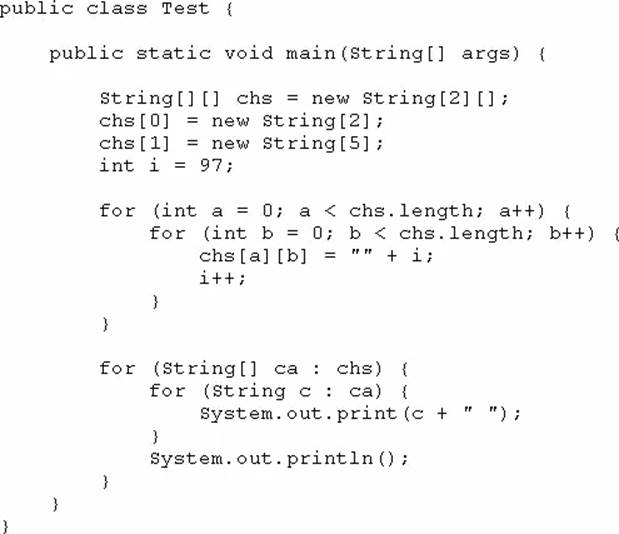25. Given the code fragment: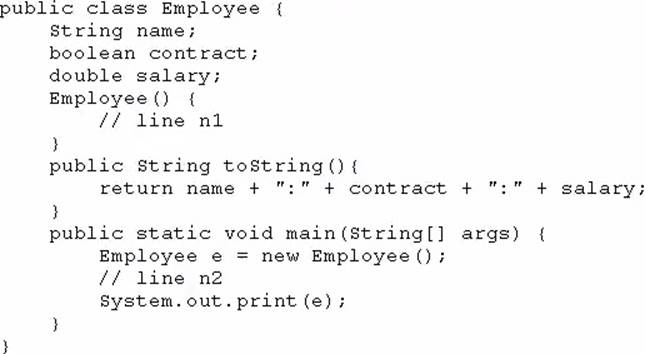Which two modifications, when made independently, enable the code to print Joe:true: 100.0? (Choose two.)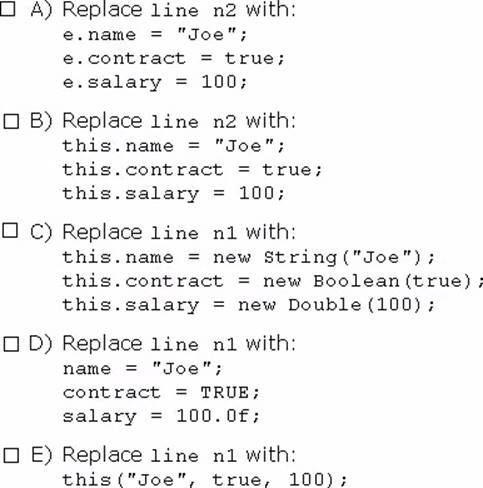26. Given the code fragment: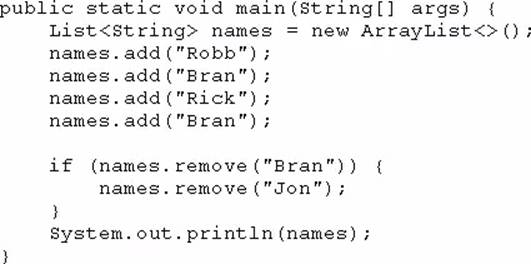What is the result?

27. Given: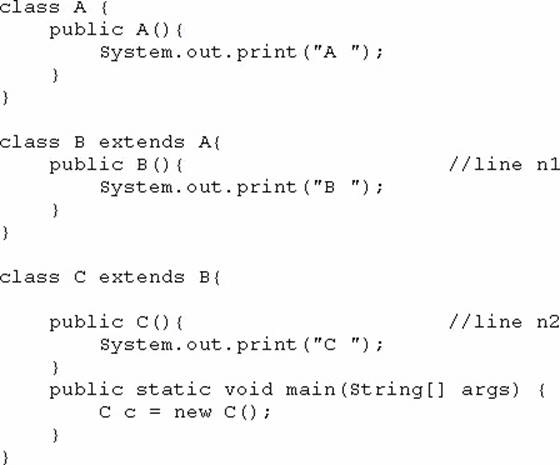What is the result?

28. Given: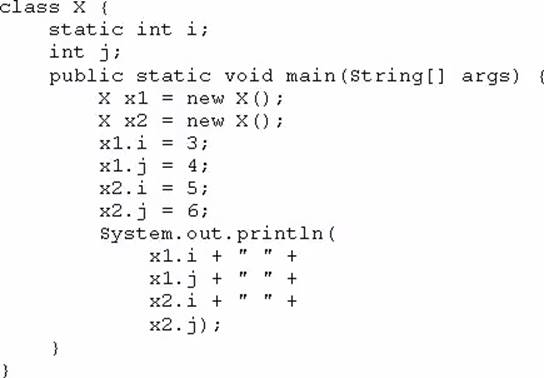What is the result?

29. Given the code fragment: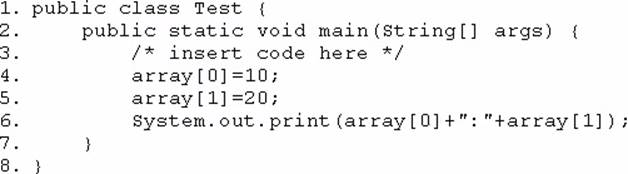Which code fragment, when inserted at line 3, enables the code to print 10:20?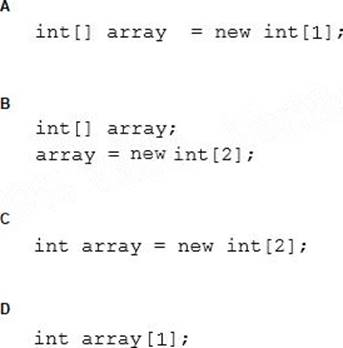30. Given the code fragment: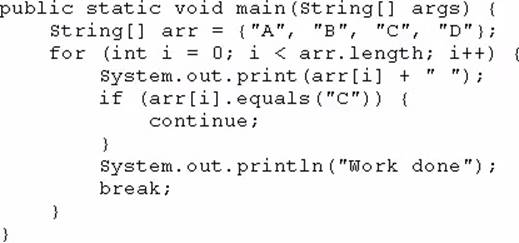What is the result?

31. Which three are advantages of the Java exception mechanism? (Choose three.)

32. Given the code from the Greeting.Java file: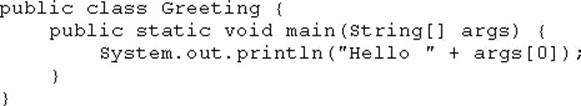Which set of commands prints Hello Duke in the console?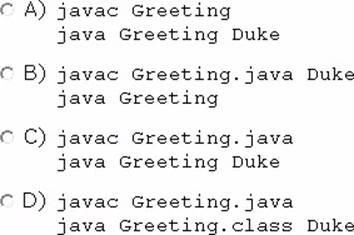33. Given: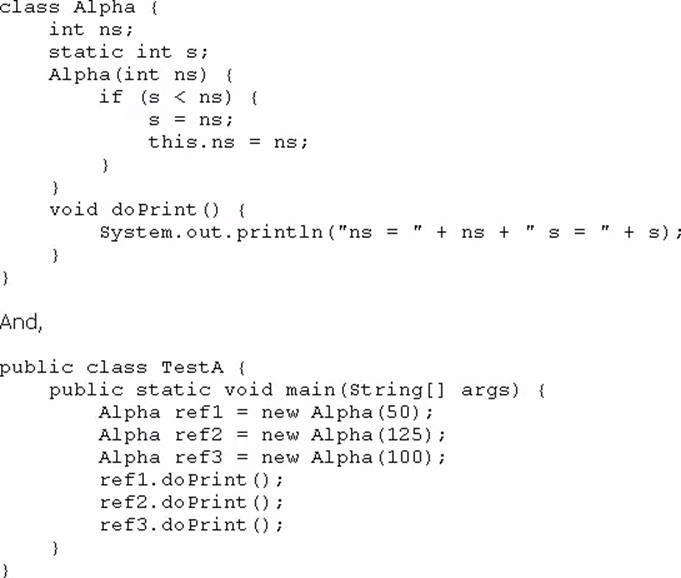What is the result?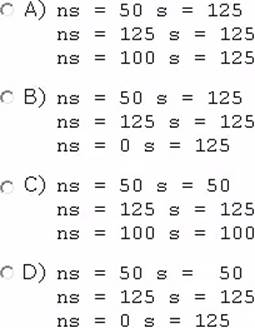34. Given the code fragment: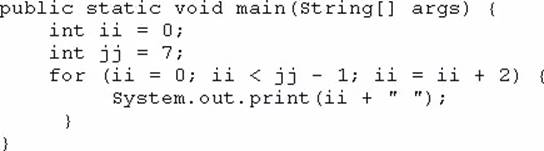What is the result?

35. Given the code fragment: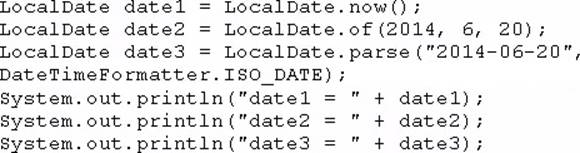Assume that the system date is June 20, 2014.

What is the result?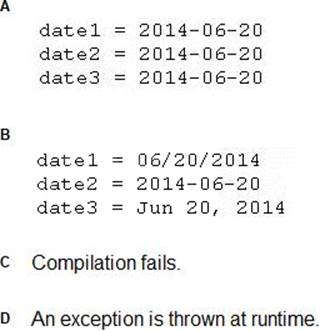36. Given the code fragment: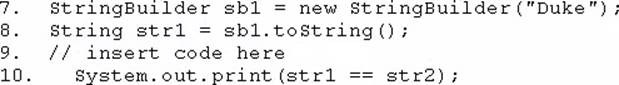Which code fragment, when inserted at line 9, enables the code to print true?

37. Given: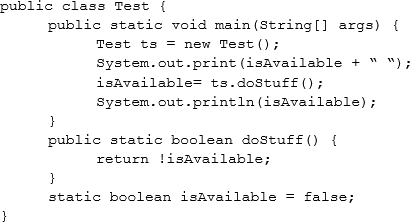What is the result?

38. Given the code fragment: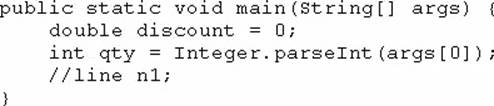And given the requirements: If the value of the qty variable is greater than or equal to 90, discount = 0.5 If the value of the qty variable is between 80 and 90, discount = 0.2

Which two code fragments can be independently placed at line n1 to meet the requirements? (Choose two.)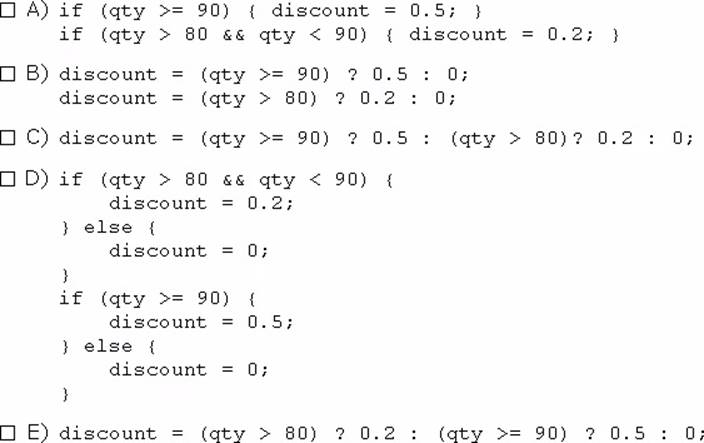39. Given: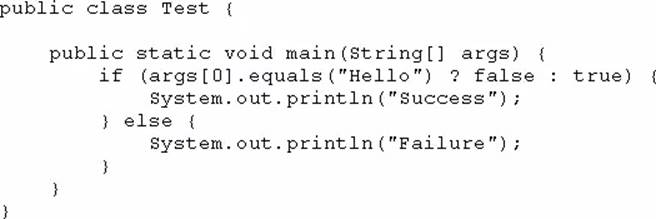And given the commands: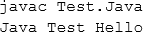What is the result?

40. Which three statements describe the object-oriented features of the Java language? (Choose three.)

41. Given the following code: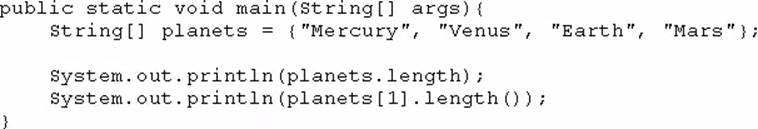What is the output?

42. You are developing a banking module. You have developed a class named ccMask that has a maskcc method.

Given the code fragment: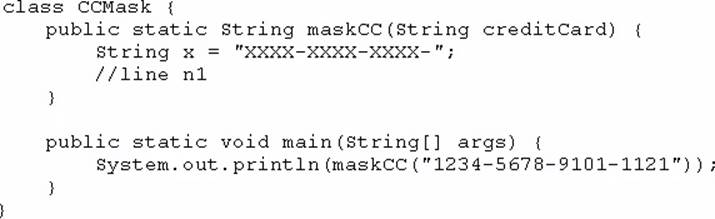You must ensure that the maskcc method returns a string that hides all digits of the credit card number except the four last digits (and the hyphens that separate each group of four digits).

Which two code fragments should you use at line n1, independently, to achieve this requirement? (Choose two.)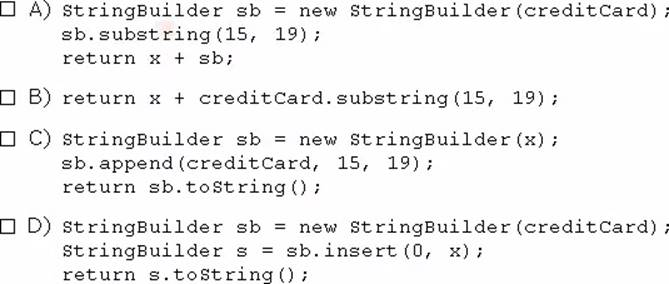43. Given: Acc.java: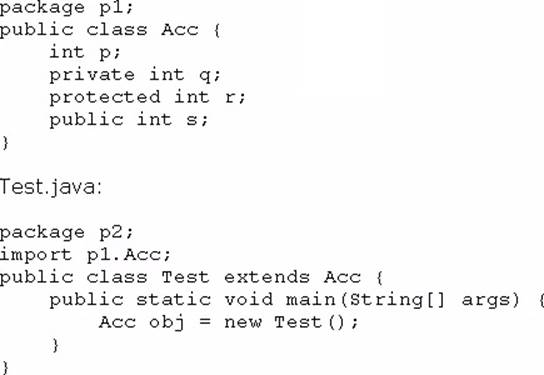Which statement is true?

44. Given: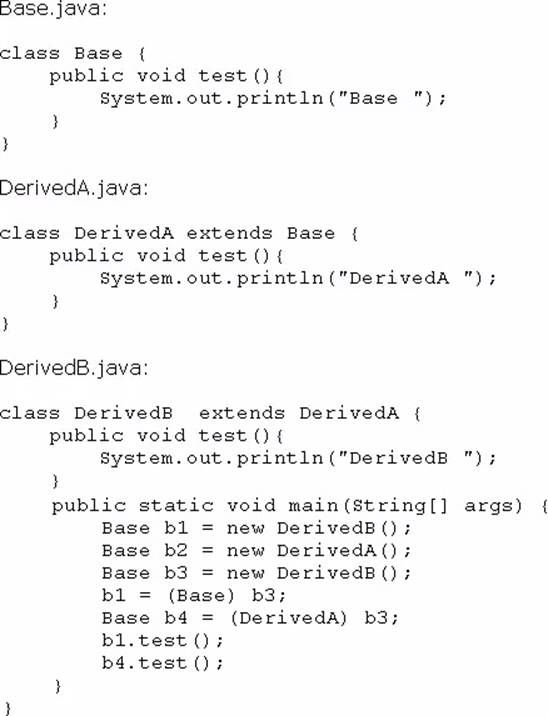What is the result?

45. Given the code fragment: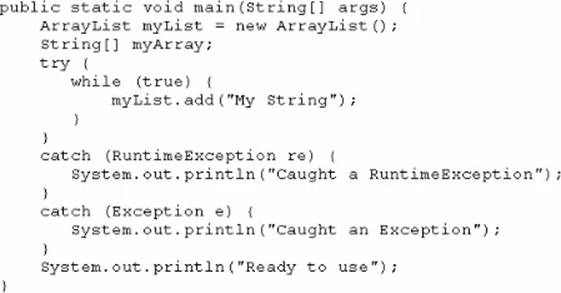What is the result?

46. Given: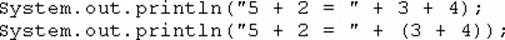What is the result?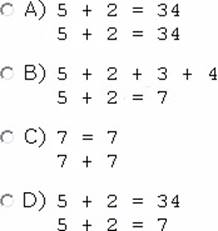47. Given the code fragments: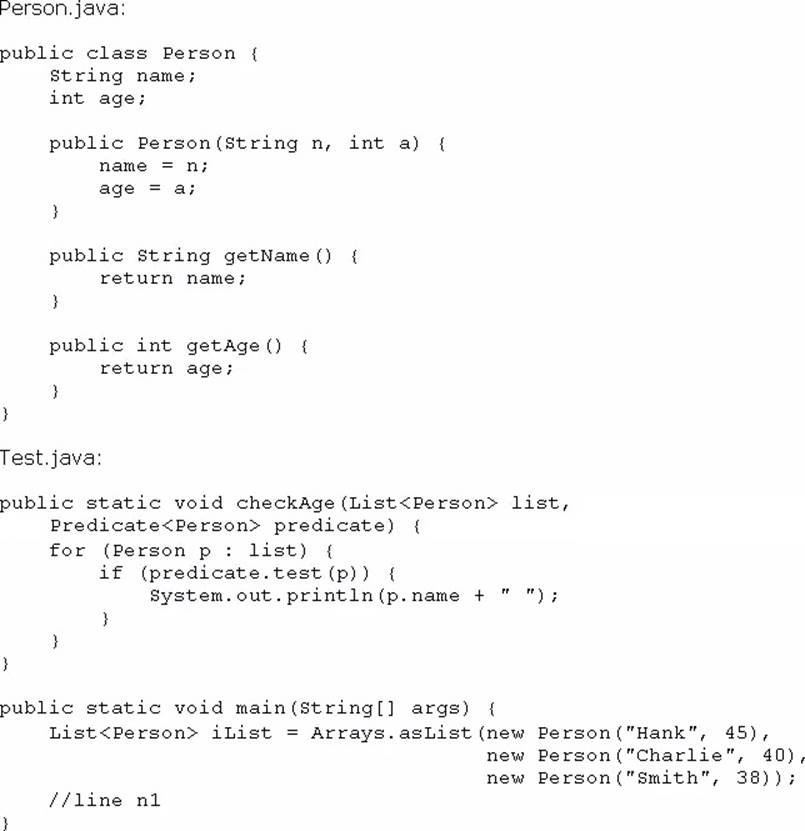Which code fragment, when inserted at line n1, enables the code to print Hank?

48. Given the code fragment: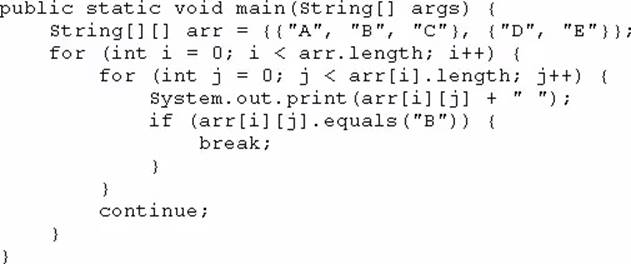What is the result?

49. Given the code fragment: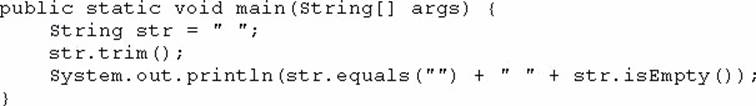What is the result?

50. Given the code fragment: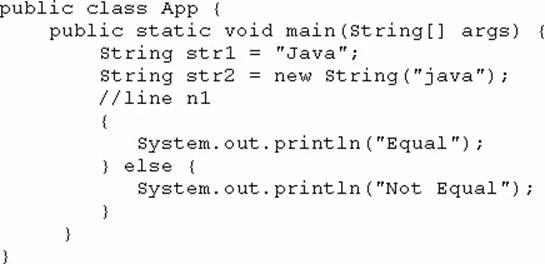Which code fragment, when inserted at line n1, enables the App class to print Equal?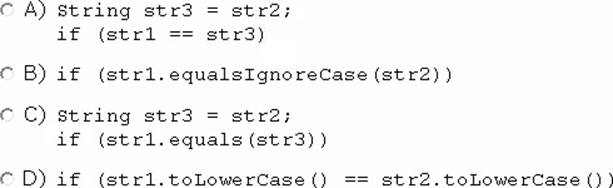51. Given the code fragment: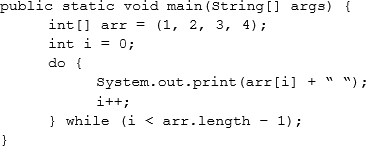What is the result?

52. Given the code fragment: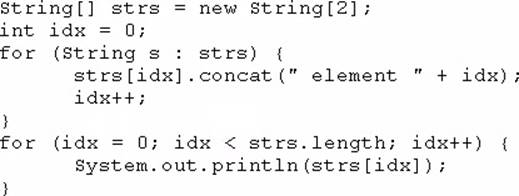What is the result?

53. Given: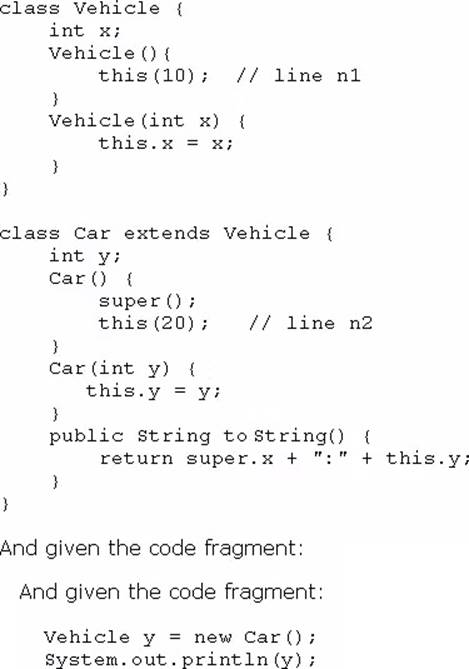What is the result?

54. Given the definitions of the MyString class and the Test class: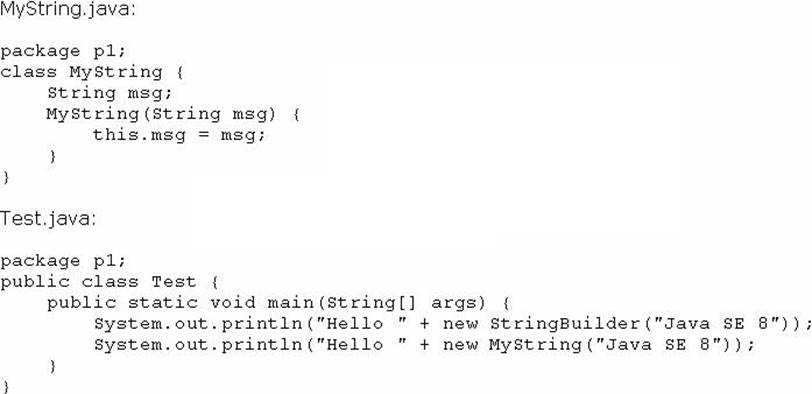What is the result?

55. Given the code fragment: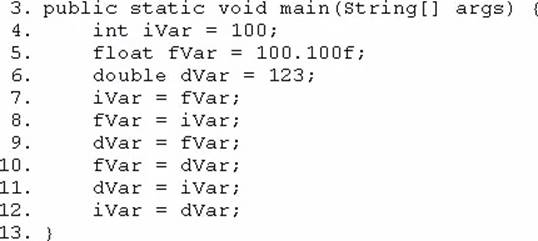Which three lines fail to compile? (Choose three.)

56. Given: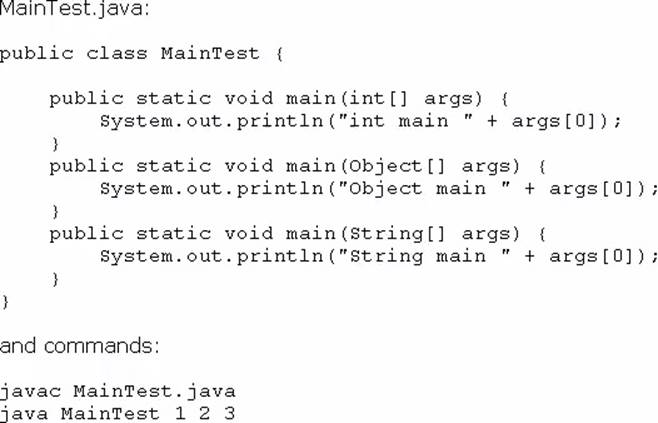What is the result?

57. Given the code fragment: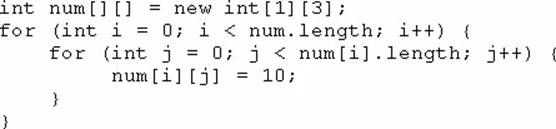Which option represents the state of the num array after successful completion of the outer loop?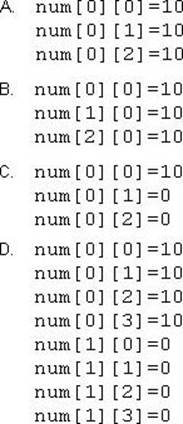58. Given this code for a Planet object: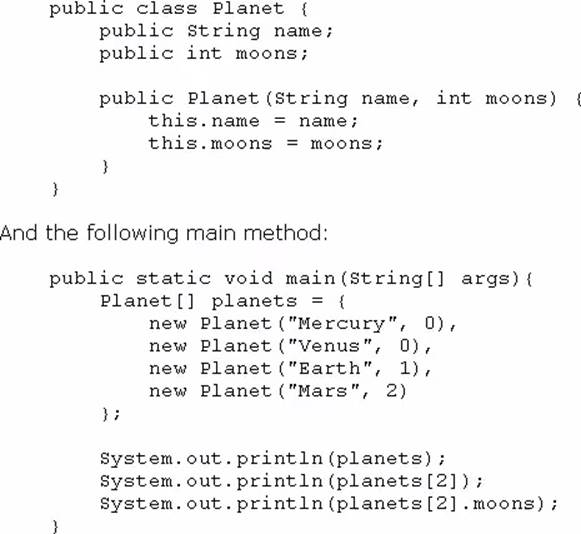What is the output?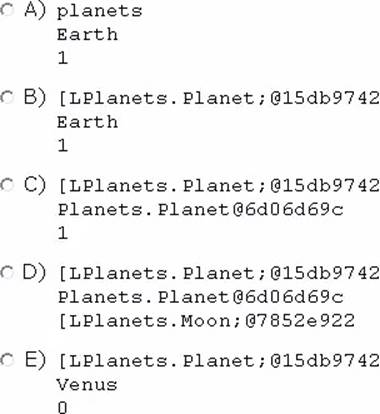59. You are asked to develop a program for a shopping application, and you are given this information:

– The application must contain the classes Toy, EduToy, and ConsToy. The Toy class is the superclass of the other two classes.

– The int calculatePrice (Toy t) method calculates the price of a toy.

– The void printToy (Toy t) method prints the details of a toy.

Which definition of the Toy class adds a valid layer of abstraction to the class hierarchy?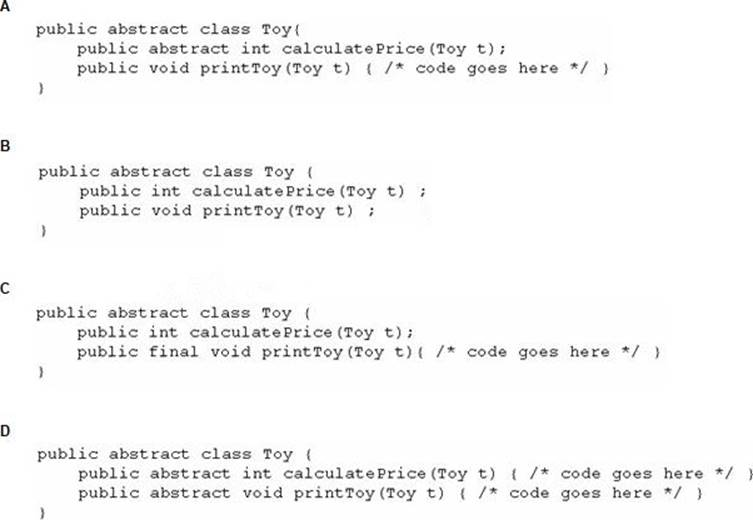60. Given the following code: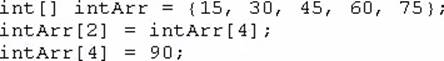What are the values of each element in intArr after this code has executed?

61. Given the content of three files: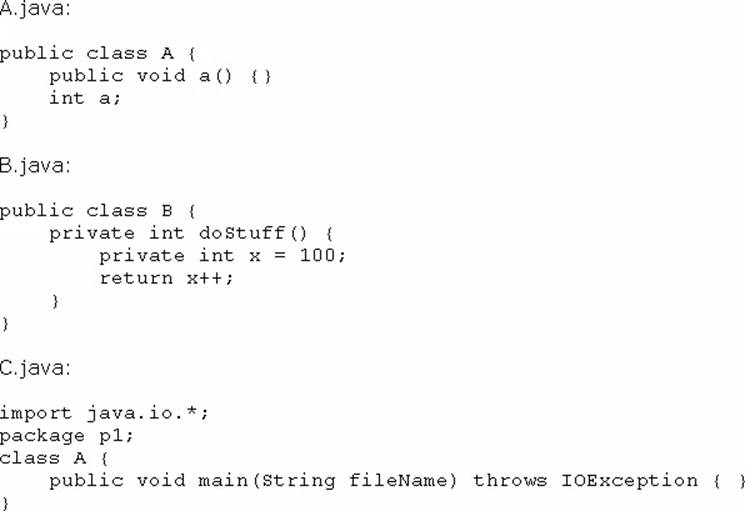Which statement is true?

62. Process alternating elements of the array in the order of entry.

Which two statements are true? (Choose two.)

63. Given: What is the result?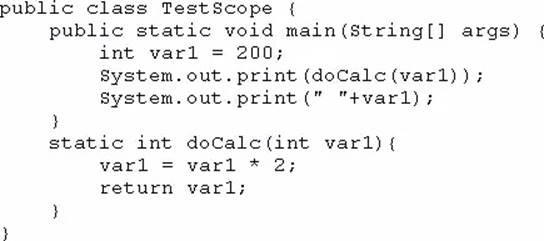64. Given the following class declarations:

– public abstract class Animal

– public interface Hunter

– public class Cat extends Animal implements Hunter

– public class Tiger extends Cat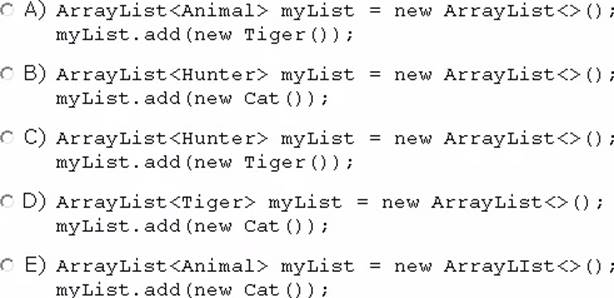65. Which statement is true about Java byte code?

66. Given: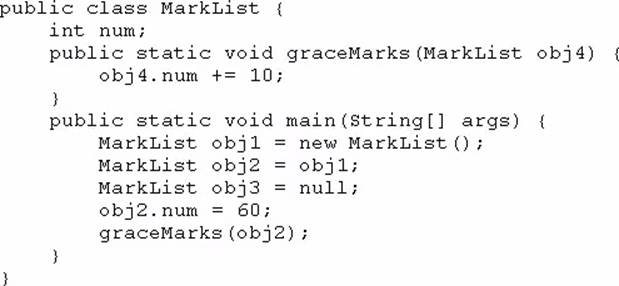How many MarkList instances are created in memory at runtime?

67. Given: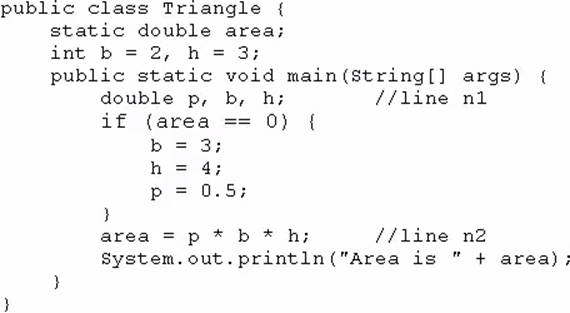What is the result?

68. Given the code fragment: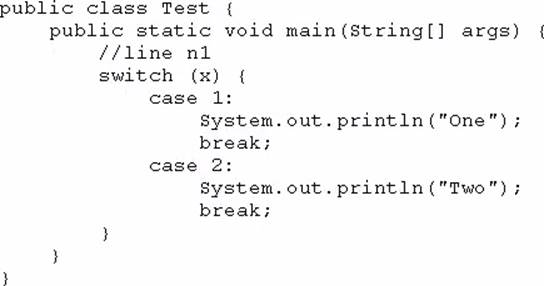Which three code fragments can be independently inserted at line n1 to enable the code to print One? (Choose three.)

69. Given: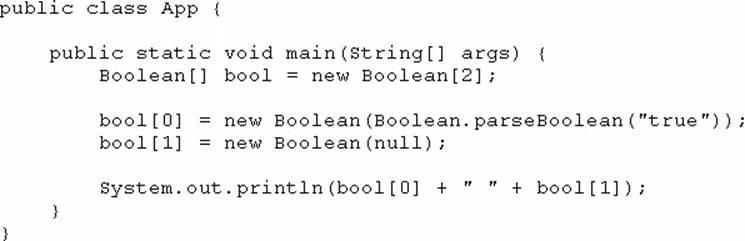What is the result?